# PSAT Math : How to find the volume of a polyhedron

## Example Questions

### Example Question #1 : How To Find The Volume Of A Polyhedron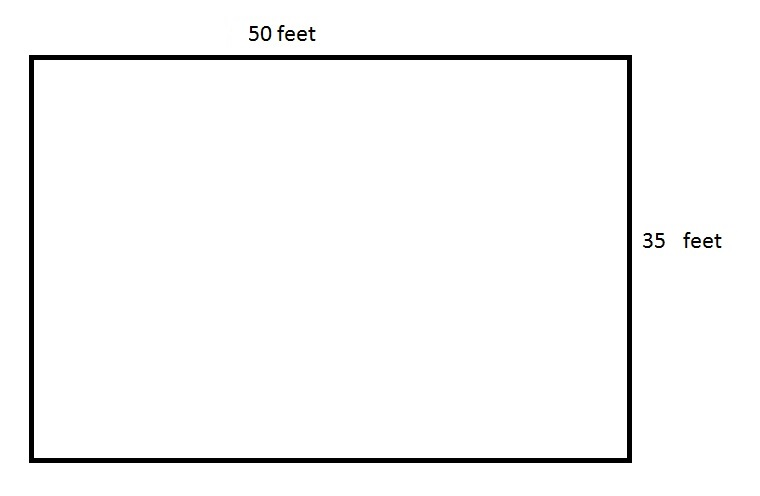The above depicts a rectangular swimming pool for an apartment. 80% of the pool is six feet deep, and the remaining part of the pool is four feet deep. How many cubic feet of water does the pool hold?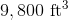None of the other choices gives the correct answer.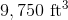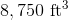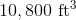Explanation:

The cross-section of the pool is the area of its surface, which is the product of its length and its width: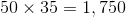square feet.

Since 80% of the pool is six feet deep, this portion of the pool holds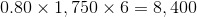cubic feet of water.

Since the remainder of the pool - 20% - is four feet deep, this portion of the pool holds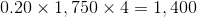cubic feet of water.

Add them together: the pool holds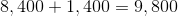cubic feet of water.